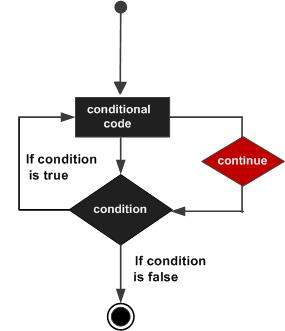# PL/SQL - CONTINUE Statement

The CONTINUE statement causes the loop to skip the remainder of its body and immediately retest its condition prior to reiterating. In other words, it forces the next iteration of the loop to take place, skipping any code in between.

## Syntax

The syntax for a CONTINUE statement is as follows −

```CONTINUE;
```

## Flow Diagram## Example

```DECLARE
a number(2) := 10;
BEGIN
-- while loop execution
WHILE a < 20 LOOP
dbms_output.put_line ('value of a: ' || a);
a := a + 1;
IF a = 15 THEN
-- skip the loop using the CONTINUE statement
a := a + 1;
CONTINUE;
END IF;
END LOOP;
END;
/
```

When the above code is executed at the SQL prompt, it produces the following result −

```value of a: 10
value of a: 11
value of a: 12
value of a: 13
value of a: 14
value of a: 16
value of a: 17
value of a: 18
value of a: 19

PL/SQL procedure successfully completed.
```
plsql_loops.htm